# allcycles

## 语法

``cycles = allcycles(G)``
``[cycles,edgecycles] = allcycles(G)``
``[___] = allcycles(G,Name,Value)``

## 说明

``cycles = allcycles(G)` 返回指定的图中的所有循环。输出 `cycles` 是元胞数组，其中每个元胞 `cycles{k}` 的内容列出形成一个循环的节点。`

``[cycles,edgecycles] = allcycles(G)` 还返回每个循环中的边。输出 `edgecycles` 是元胞数组，其中 `edgecycles{k}` 给出对应循环 `cycles{k}` 中的边。`

``[___] = allcycles(G,Name,Value)` 使用一个或多个名称-值参数指定其他选项。您可以使用上述语法中的任何输出参数组合。例如，您可以指定 `MaxNumCycles` 和一个标量来限制返回的循环数。`

## 示例

```s = [1 2 3 6 5 5 4 6 9 8 8 7]; t = [2 3 6 5 2 4 1 9 8 5 7 4]; G = digraph(s,t); plot(G)```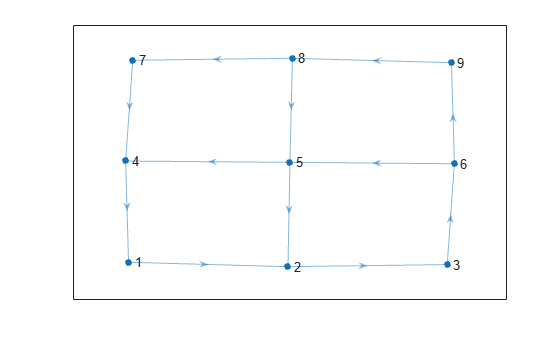`cycles = allcycles(G)`
```cycles=5×1 cell array {[ 1 2 3 6 5 4]} {[1 2 3 6 9 8 5 4]} {[1 2 3 6 9 8 7 4]} {[ 2 3 6 5]} {[ 2 3 6 9 8 5]} ```

`allcycles` 的第二个输出参数返回每个循环中包含的边。这对于多重图特别有用，在多重图中，需要边索引来唯一标识每个循环中的边。

```s = [1 1 2 2 3 3 2 2 4 6 8 6 6 7 3 3 5 3]; t = [2 3 1 3 2 1 4 4 6 2 6 7 8 8 5 5 7 7]; names = {'A','B','C','D','E','F','G','H'}; G = digraph(s,t,[],names); p = plot(G,'EdgeLabel',1:numedges(G));```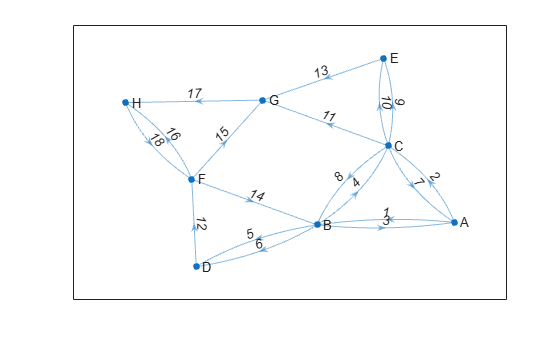`[cycles,edgecycles] = allcycles(G);`

`cycles{5}`
```ans = 1x7 cell {'A'} {'C'} {'E'} {'G'} {'H'} {'F'} {'B'} ```
`edgecycles{5}`
```ans = 1×7 2 9 13 17 18 14 3 ```

`highlight(p,'Edges',edgecycles{5},'EdgeColor','r','LineWidth',1.5,'NodeColor','r','MarkerSize',6)`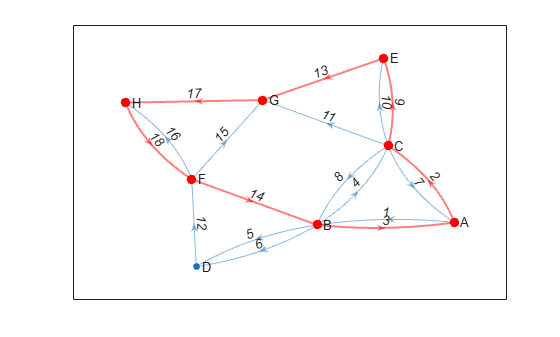```A = ones(20); G = graph(A,'omitselfloops');```

`cycles1 = allcycles(G,'MaxNumCycles',10)`
```cycles1=10×1 cell array {[ 1 2 3]} {[ 1 2 3 4]} {[ 1 2 3 4 5]} {[ 1 2 3 4 5 6]} {[ 1 2 3 4 5 6 7]} {[ 1 2 3 4 5 6 7 8]} {[ 1 2 3 4 5 6 7 8 9]} {[ 1 2 3 4 5 6 7 8 9 10]} {[ 1 2 3 4 5 6 7 8 9 10 11]} {[1 2 3 4 5 6 7 8 9 10 11 12]} ```

`cycles2 = allcycles(G,'MaxNumCycles',10,'MaxCycleLength',3)`
```cycles2=10×1 cell array {[ 1 2 3]} {[ 1 2 4]} {[ 1 2 5]} {[ 1 2 6]} {[ 1 2 7]} {[ 1 2 8]} {[ 1 2 9]} {[1 2 10]} {[1 2 11]} {[1 2 12]} ```

`cycles3 = allcycles(G,'MaxNumCycles',10,'MinCycleLength',4)`
```cycles3=10×1 cell array {[ 1 2 3 4]} {[ 1 2 3 4 5]} {[ 1 2 3 4 5 6]} {[ 1 2 3 4 5 6 7]} {[ 1 2 3 4 5 6 7 8]} {[ 1 2 3 4 5 6 7 8 9]} {[ 1 2 3 4 5 6 7 8 9 10]} {[ 1 2 3 4 5 6 7 8 9 10 11]} {[ 1 2 3 4 5 6 7 8 9 10 11 12]} {[1 2 3 4 5 6 7 8 9 10 11 12 13]} ```

```n = 5; A = delsq(numgrid('S',n)); G = graph(A,'omitselfloops'); plot(G)```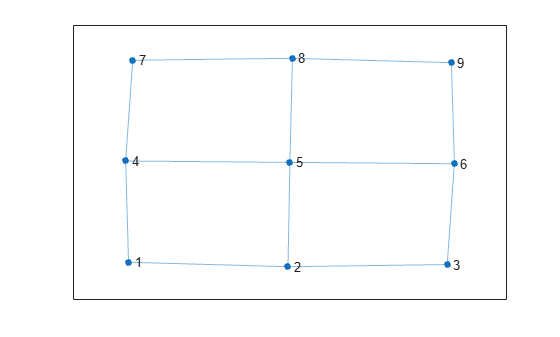```[cycles,edgecycles] = allcycles(G); tiledlayout flow for k = 1:length(cycles) nexttile highlight(plot(G),cycles{k},'Edges',edgecycles{k},'EdgeColor','r','NodeColor','r') title("Cycle " + k) end```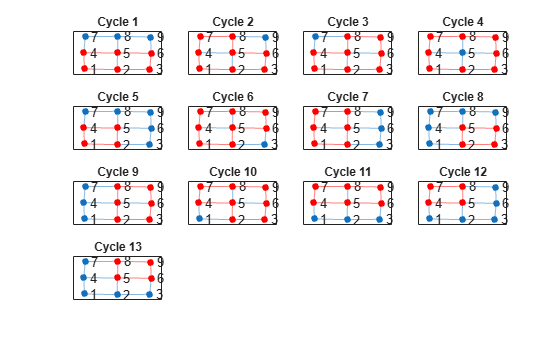```[cycles,edgecycles] = cyclebasis(G); tiledlayout flow for k = 1:length(cycles) nexttile highlight(plot(G),cycles{k},'Edges',edgecycles{k},'EdgeColor','r','NodeColor','r') title("Cycle " + k) end```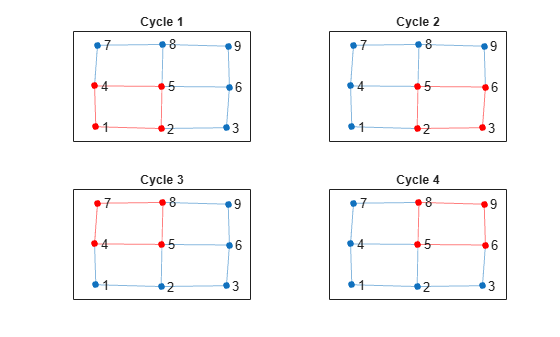```n = 6; A = delsq(numgrid('S',n)); G = graph(A,'omitselfloops'); figure plot(G)```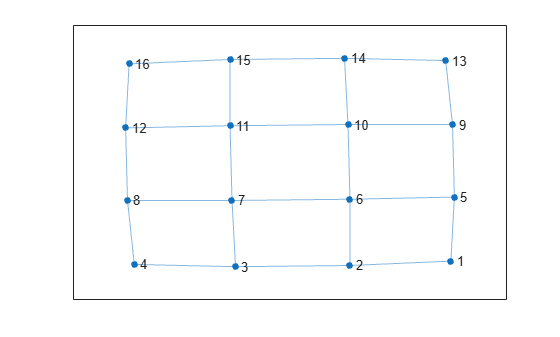`allcycles(G)`
```ans=213×1 cell array {[ 1 2 3 4 8 7 6 5]} {[ 1 2 3 4 8 7 6 10 9 5]} {[1 2 3 4 8 7 6 10 11 12 16 15 14 13 9 5]} {[ 1 2 3 4 8 7 6 10 11 15 14 13 9 5]} {[ 1 2 3 4 8 7 6 10 14 13 9 5]} {[ 1 2 3 4 8 7 11 10 6 5]} {[ 1 2 3 4 8 7 11 10 9 5]} {[ 1 2 3 4 8 7 11 10 14 13 9 5]} {[ 1 2 3 4 8 7 11 12 16 15 14 10 6 5]} {[ 1 2 3 4 8 7 11 12 16 15 14 10 9 5]} {[ 1 2 3 4 8 7 11 12 16 15 14 13 9 5]} {[1 2 3 4 8 7 11 12 16 15 14 13 9 10 6 5]} {[ 1 2 3 4 8 7 11 15 14 10 6 5]} {[ 1 2 3 4 8 7 11 15 14 10 9 5]} {[ 1 2 3 4 8 7 11 15 14 13 9 5]} {[ 1 2 3 4 8 7 11 15 14 13 9 10 6 5]} ⋮ ```

```[cycles,edgecycles] = cyclebasis(G); figure tiledlayout flow for k = 1:length(cycles) nexttile highlight(plot(G),cycles{k},'Edges',edgecycles{k},'EdgeColor','r','NodeColor','r') title("Cycle " + k) end```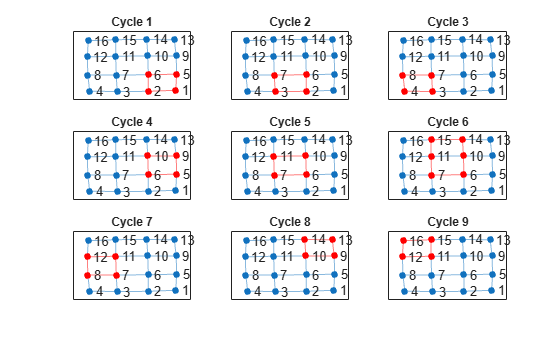## 输出参数

`cycles` 中元胞的数据类型取决于输入图是否包含节点名称：

• 如果图 `G` 没有节点名称，则每个元素 `cycles{k}` 均为节点索引的数值向量。

• 如果图 `G` 有节点名称，则每个元素 `cycles{k}` 均为字符向量节点名称的元胞数组。

## 提示

• 图中的循环数在很大程度上取决于图的结构。对于某些图结构，循环数可能随着节点数增加而呈指数增长。例如，一个具有由 `G = graph(ones(12))` 给出的 12 个节点的完整图包含近 6000 万个循环。在这些情况下，请使用 `MaxNumCycles``MaxCycleLength``MinCycleLength` 选项来控制 `allcycles` 的输出。